#### You may also like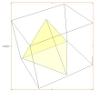### A Mean Tetrahedron

Can you number the vertices, edges and faces of a tetrahedron so that the number on each edge is the mean of the numbers on the adjacent vertices and the mean of the numbers on the adjacent faces?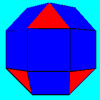### Rhombicubocts

Each of these solids is made up with 3 squares and a triangle around each vertex. Each has a total of 18 square faces and 8 faces that are equilateral triangles. How many faces, edges and vertices does each solid have?### Triangles to Tetrahedra

Imagine you have an unlimited number of four types of triangle. How many different tetrahedra can you make?

# Icosian Game

##### Age 11 to 14Challenge Level

For the tetrahedron, I have:

I tested all Platonic solids: the tetrahedron, the cube, the octahedron, the dodecahedron and the icosahedron.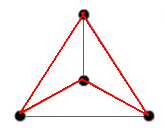For the tetrahedron, there was simple - there were only four points to study. I obtained more possible arrangements, corresponding to different solutions. The other, not shown is to go on the outside circuit up to the last-but-one point, than to go to the centre and finally at the starting point. There are different solutions only if the start - finish is represented on the figure, otherwise this solution is obtained from the first by a rotation.

For the cube, I worked on an applet: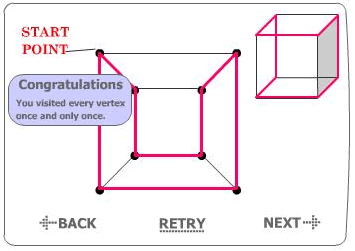The strategy I used is the following: I go on the outer lines, up to the last-but-one point. Then I go through the inner one. I can use symmetrical combinations, obtaining more than one path.

Looking at the octahedron, I take the same strategy as for the cube => there are many combinations.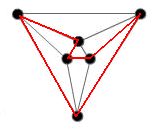For the dodecahedron, I worked on an applet, taking the same strategy as for the cube, the difference is that here there are more layers.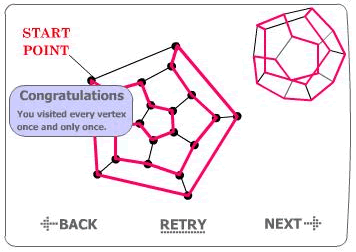The last polyhedron I test is the icosahedron: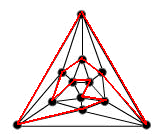Here, I worked in the same manner as before, obtaining the figure shown. There are naturally more combinations.